# All You Need to Know to get started with NumPyEverything you need to know to get started with NumPy. The Ultimate Beginner’s Guide to NumPy

The world runs on data and everyone should know how to work with it.

It’s hard to imagine a modern, tech-literate business that doesn’t use data analysis, data science, machine learning, or artificial intelligence in some form. NumPy is at the core of all of those fields.

While it’s impossible to know exactly how many people are learning to analyze and work with data, it’s a pretty safe assumption that tens of thousands (if not millions) of people need to understand NumPy and how to use it. Because of that, I’ve spent the last three months putting together what I hope is the best introductory guide to NumPy yet! If there’s anything you want to see included in this tutorial, please leave a note in the comments or reach out any time!

If you’re new to data analysis, this one’s for you.

## The Absolute Beginner’s Guide to NumPy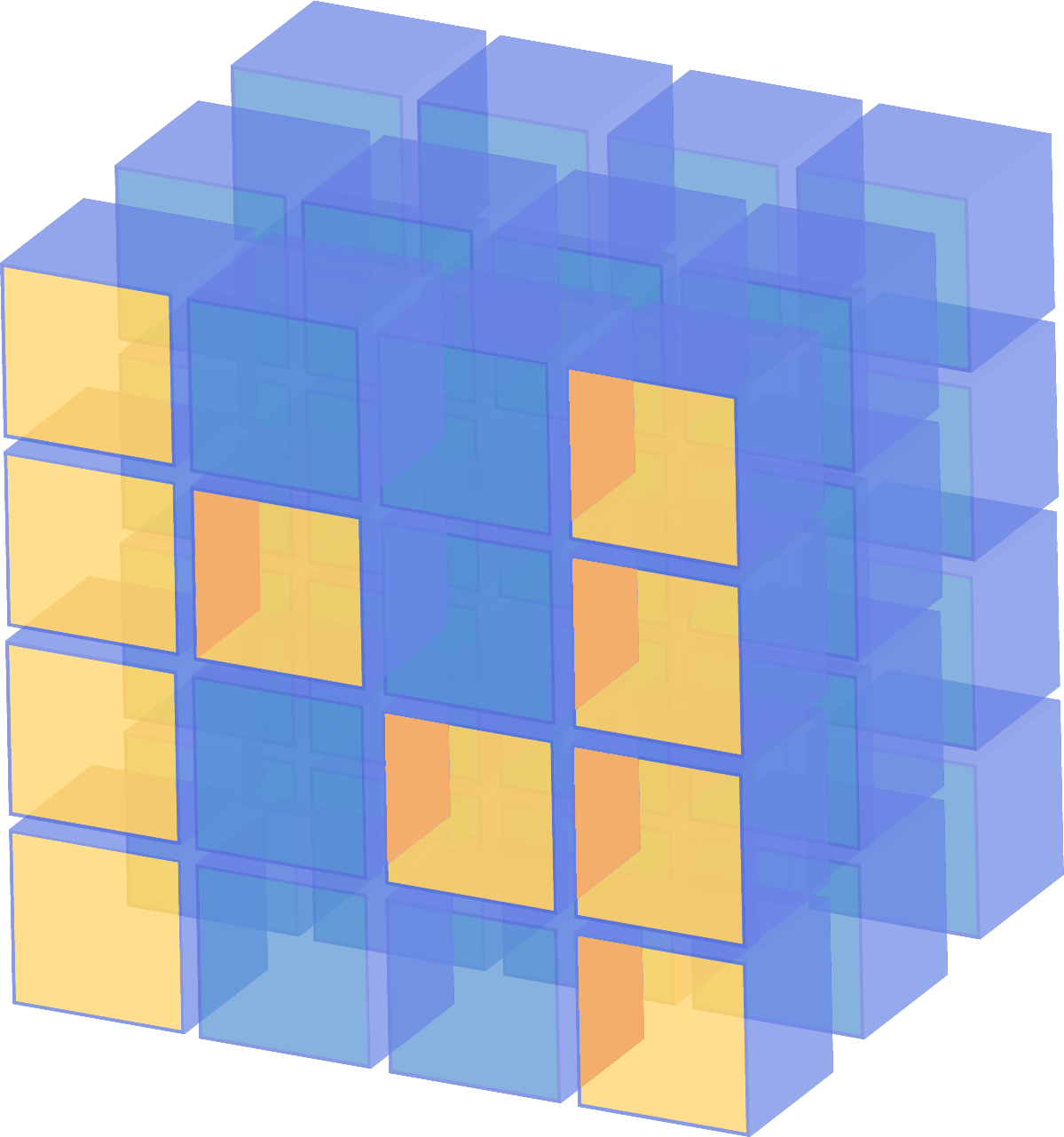### Welcome to NumPy!

NumPy (Numerical Python) is an open-source Python library that’s used in almost every field of science and engineering. It’s the universal standard for working with numerical data in Python, and it’s at the core of the scientific Python and PyData ecosystems. NumPy users include everyone from beginning coders to experienced researchers doing state-of-the-art scientific and industrial research and development. The NumPy API is used extensively in Pandas, SciPy, Matplotlib, scikit-learn, scikit-image and most other data science and scientific Python packages.

The NumPy library contains multidimensional array and matrix data structures (you’ll find more information about this in later sections). It provides ndarray, a homogeneous n-dimensional array object, with methods to efficiently operate on it. NumPy can be used to perform a wide variety of mathematical operations on arrays. It adds powerful data structures to Python that guarantee efficient calculations with arrays and matrices and it supplies an enormous library of high-level mathematical functions that operate on these arrays and matrices.

## Installing NumPy

To install NumPy, I strongly recommend using a scientific Python distribution. If you’re looking for the full instructions for installing NumPy on your operating system, you can find all of the details here.

If you already have Python, you can install NumPy with

``conda install numpy``

or

``pip install numpy``

If you don’t have Python yet, you might want to consider using Anaconda. It’s the easiest way to get started. The good thing about getting this distribution is the fact that you don’t need to worry too much about separately installing NumPy or any of the major packages that you’ll be using for your data analyses, like pandas, Scikit-Learn, etc.

If you need more details about installation, you can find all of the installation information at scipy.org.## How to import NumPy

Any time you want to use a package or library in your code, you first need to make it accessible.

In order to start using NumPy and all of the functions available in NumPy, you’ll need to import it. This can be easily done with this import statement:

``import numpy as np``

(We shorten “numpy” to “np” in order to save time and also to keep code standardized so that anyone working with your code can easily understand and run it.)

## What’s the difference between a Python list and a NumPy array?

NumPy gives you an enormous range of fast and efficient numerically-related options. While a Python list can contain different data types within a single list, all of the elements in a NumPy array should be homogenous. The mathematical operations that are meant to be performed on arrays wouldn’t be possible if the arrays weren’t homogenous.

Why use NumPy?NumPy arrays are faster and more compact than Python lists. An array consumes less memory and is far more convenient to use. NumPy uses much less memory to store data and it provides a mechanism of specifying the data types, which allows the code to be optimized even further.

## What is an array?

An array is a central data structure of the NumPy library. It’s a grid of values and it contains information about the raw data, how to locate an element, and how to interpret an element. It has a grid of elements that can be indexed in various ways. The elements are all of the same type, referred to as the array dtype (data type).

An array can be indexed by a tuple of nonnegative integers, by booleans, by another array, or by integers. The rank of the array is the number of dimensions. The shape of the array is a tuple of integers giving the size of the array along each dimension.

One way we can initialize NumPy arrays is from nested Python lists.

``a = np.array([[1 , 2, 3, 4], [5, 6, 7, 8], [9, 10, 11, 12]])``

We can access the elements in the array using square brackets. When you’re accessing elements, remember that indexing in NumPy starts at 0. That means that if you want to access the first element in your array, you’ll be accessing element “0”.

``print(a)``

Output:

``[1 2 3 4]``

``1D array, 2D array, ndarray, vector, matrix``

You might occasionally hear an array referred to as a “ndarray,” which is shorthand for “N-dimensional array.” An N-dimensional array is simply an array with any number of dimensions. You might also hear 1-D, or one-dimensional array, 2-D, or two-dimensional array, and so on. The NumPy ndarray class is used to represent both matrices and vectors. A vector is an array with a single column, while a matrix refers to an array with multiple columns.

What are the attributes of an array?

An array is usually a fixed-size container of items of the same type and size. The number of dimensions and items in an array is defined by its shape. The shape of an array is a tuple of non-negative integers that specify the sizes of each dimension.

In NumPy, dimensions are called axes. This means that if you have a 2D array that looks like this:

``````[[0., 0., 0.],
[1., 1., 1.]]``````

Your array has 2 axes. The first axis has a length of 2 and the second axis has a length of 3.

Just like in other Python container objects, the contents of an array can be accessed and modified by indexing or slicing the array. Different arrays can share the same data, so changes made on one array might be visible in another.

Array attributes reflect information intrinsic to the array itself. If you need to get, or even set, properties of an array without creating a new array, you can often access an array through its attributes.

## How to create a basic array

``````np.array()
np.zeros()
np.ones()
np.empty()
np.arange()
np.linspace()
dtype``````

To create a NumPy array, you can use the function np.array().

All you need to do to create a simple array is pass a list to it. If you choose to, you can also specify the type of data in your list. You can find more information about data types here.

``import numpy as npa = np.array([1, 2, 3])``

You can visualize your array this way:Besides creating an array from a sequence of elements, you can easily create an array filled with 0s:

Input:

``np.zeros(2``

Output:

``array([0., 0.])``

Or an array filled with 1s:

Input:

``np.ones(2)``

Output:

``array([1., 1.])``

Or even an empty array! The function empty creates an array whose initial content is random and depends on the state of the memory.

Input:

``````# Create an empty array with 2 elements
np.empty(2)``````

You can create an array with a range of elements:

Input:

``np.arange(4)``

Output:

``array([0, 1, 2, 3])``

And even an array that contains a range of evenly spaced intervals. To do this, you will specify the first number, last number, and the step size.

Input:

``np.arange(2,9,2)``

Output:

``array([2, 4, 6, 8])``

You can also use np.linspace() to create an array with values that are spaced linearly in a specified interval:

Input:

``np.linspace(0,10,5)``

Output:

``array([ 0. ,  2.5,  5. ,  7.5, 10. ])``

While the default data type is floating point (float64), you can explicitly specify which data type you want using dtype.

Input:

``````array = np.ones(2, dtype=int)
array``````

Output:

``array([1, 1])``

Learn more about creating arrays here.## Adding, removing, and sorting elements

``````np.append()
np.delete()
np.sort()``````

``arr = np.array([1, 2, 3, 4, 5, 6, 7, 8])``

You can add elements to your array any time with np.append(). Make sure to specify the array and the elements you want to include.

Input:

``np.append(arr, [1,2])``

Output:

``array([1, 2, 3, 4, 5, 6, 7, 8, 1, 2])``

You can delete an element with np.delete(). If you want to delete the element in position 1 of your array, you can run:

Input:

``np.delete(arr, 1)``

Output:

``array([1, 3, 4, 5, 6, 7, 8])``

Sorting an element is simple with np.sort(). You can specify the axis, kind, and order when you call the function. Read more about sorting an array here.

``arr = np.array([2, 1, 5, 3, 7, 4, 6, 8])``

You can quickly sort the numbers in ascending order with:

Input:

``np.sort(arr)``

Output:

``array([1, 2, 3, 4, 5, 6, 7, 8])``

In addition to sort, which returns a sorted copy of an array, you can use: argsort, which is an indirect sort along a specified axis, lexsort, which is an indirect stable sort on multiple keys, searchsorted, which will find elements in a sorted array, and partition, which is a partial sort.

## How do you know the shape and size of an array?

``````ndarray.ndim()
ndarray.size()
ndarray.shape()``````

ndarray.ndim will tell you the number of axes, or dimensions, of the array.

ndarray.size will tell you the total number of elements of the array. This is the product of the elements of the array’s shape.

ndarray.shape will display a tuple of integers that indicate the number of elements stored along each dimension of the array. If, for example, you have a 2D array with 2 rows and 3 columns, the shape of your array is (2,3).

For example, if you create this array:

``````array_example = np.array([[[0, 1, 2, 3]
[4, 5, 6, 7]],                           [[0, 1, 2, 3]
[4, 5, 6, 7]],                            [0 ,1 ,2, 3]
[4, 5, 6, 7]]])``````

To find the number of dimensions of the array, run:

Input:

``array_example.ndim``

Output:

``3``

To find the total number of elements in the array, run:

Input:

``array_example.size``

Output:

``24``

And to find the shape of your array, run:

Input:

``array_example.shape``

Output:

``(3,2,4)``

Using np.reshape() will give a new shape to an array without changing the data. Just remember that when you use the reshape method, the array you want to produce needs to have the same number of elements as the original array. If you start with an array with 12 elements, you’ll need to make sure that your new array also has a total of 12 elements.

``````a = np.arange(6)
print(a)``````

Output:

``[0 1 2 3 4 5]``

You can use reshape() to reshape your array. For example, you can reshape this array to an array with three rows and two columns:

Input:

``````b = a.reshape(3,2)
print(b)``````

Output:

``````[[0 1]
[2 3]
[4 5]]``````

With np.reshape, you can specify a few optional parameters:

Input:

``numpy.reshape(a, newshape, order)``

a is the array to be reshaped.

newshape is the new shape you want. You can specify an integer or a tuple of integers. If you specify an integer, the result will be an array of that length. The shape should be compatible with the original shape.

order: ‘C’ means to read/write the elements using C-like index order, ‘F’ means to read/write the elements using Fortran-like index order, ‘A’ means to read/write the elements in Fortran-like index order if a is Fortran contiguous in memory, C-like order otherwise. (This is an optional parameter and doesn’t need to be specified.)

## How to convert a 1D array into a 2D array (how to add a new axis to an array)

``````np.newaxis
np.expand_dims``````

You can use np.newaxis and np.expand_dims to increase the dimensions of your existing array.

Using np.newaxis will increase the dimensions of your array by one dimension when used once. This means that a 1D array will become a 2D array, a 2D array will become a 3D array, and so on.

``````a = np.array([1, 2, 3, 4, 5, 6])
a.shape``````

Output:

``(6,)``

You can use np.newaxis to add a new axis:

Input:

``````a2 = a[np.newaxis]
a2.shape``````

Output:

``(1, 6)``

You can explicitly convert a 1D array with either a row vector or a column vector using np.newaxis. For example, you can convert a 1D array to a row vector by inserting an axis along the first dimension:

Input:

``````row_vector = a[np.newaxis, :]
row_vector.shape``````

Output:

``(1, 6)``

Or, for a column vector, you can insert an axis along the second dimension:

Input:

``````col_vector = a[:, np.newaxis]
col_vector.shape``````

Output:

``(6, 1)``

You can also expand an array by inserting a new axis at a specified position with np.expand_dims.

Input:

``````a = np.array([1, 2, 3, 4, 5, 6])
a.shape``````

Output:

``(6,)``

You can use np.expand_dims to add an axis at index position 1 with:

Input:

``````b = np.expand_dims(a, axis=1)
b.shape``````

Output:

``(6, 1)``

You can add an axis at index position 0 with:

Input:

``````c = np.expand_dims(a, axis=0)
c.shape``````

Output:

``(1, 6)``

Find more information about newaxis here and expand_dims here.## Indexing and slicing

You can index and slice NumPy arrays in the same ways you can slice Python lists.

Input:

``````data = np.array([1,2,3])print(data)
print(data)
print(data[0:2])
print(data[1:])
print(data[-2:])``````

Output:

``````1
2
[1 2]
[2 3]``````

You can visualize it this way: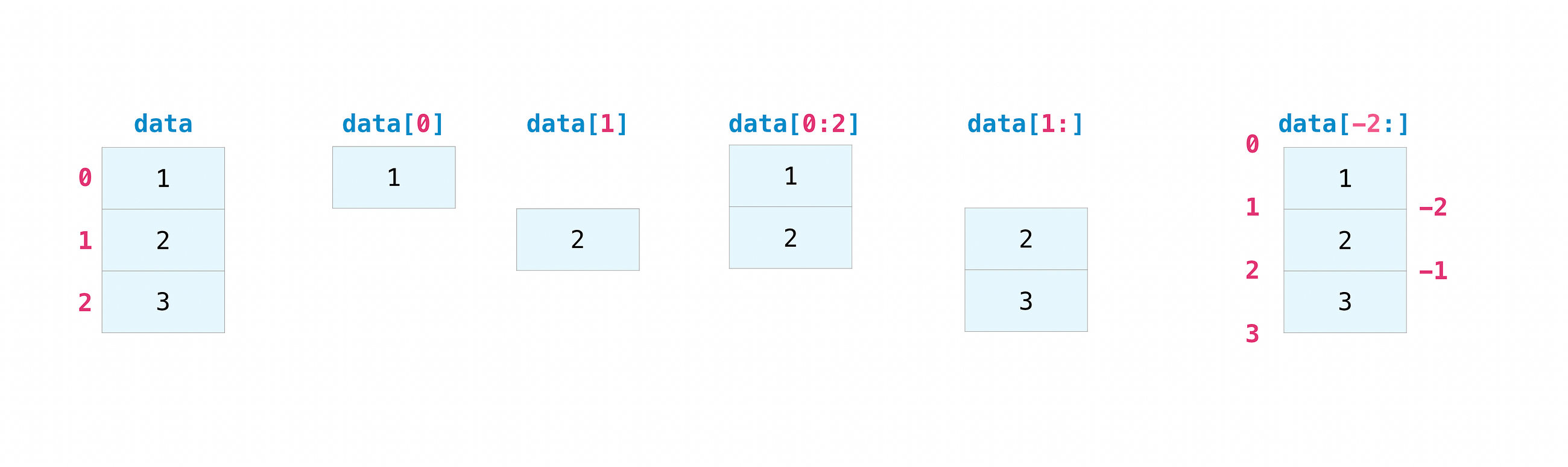You may want to take a section of your array or specific array elements to use in further analysis or additional operations. To do that, you’ll need to subset, slice, and/or index your arrays.

If you want to select values from your array that fulfill certain conditions, it’s straightforward with NumPy.

``a = np.array([[1 , 2, 3, 4], [5, 6, 7, 8], [9, 10, 11, 12]])``

You can easily print all of the values in the array that are less than 5.

Input:

``print(a[a<5])``

Output:

``[1 2 3 4]``

You can also select, for example, numbers that are equal to or greater than 5, and use that condition to index an array.

Input:

``````five_up = (a >= 5)
print(a[five_up])``````

Output:

``[ 5  6  7  8  9 10 11 12]``

You can select elements that are divisible by 2:

Input:

``````divisible_by_2 = a[a%2==0]
print(divisible_by_2)``````

Output:

``[ 2  4  6  8 10 12]``

Or you can select elements that satisfy two conditions using the & and | operators:

Input:

``````c = a[(a > 2) & (a < 11)]
print(c)``````

Output:

``[ 3  4  5  6  7  8  9 10]``

While it would be incredibly inefficient for this array, you can also make use of the logical operators & and | in order to return boolean values that specify whether or not the values in an array fulfill a certain condition. This can be useful with arrays that contain names or other categorical values.

Input:

``````five_up = (array > 5) | (array == 5)
print(five_up)``````

Output:

``````[[False False False False]
[ True  True  True  True]
[ True  True  True  True]]``````

You can also use np.where() to select elements or indices from an array.

Starting with this array:

Input:

``a = np.array([[1 , 2, 3, 4], [5, 6, 7, 8], [9, 10, 11, 12]])``

You can use np.where() to print the indices of elements that are, for example, less than 5:

Input:

``````b = np.where(a<5)
print(b)``````

Output:

``(array([0, 0, 0, 0]), array([0, 1, 2, 3]))``

In this example, a tuple of arrays was returned: one for each dimension. The first array represents the row indices where these values are found, and the second array represents the column indices where the values are found.

If you want to generate a list of coordinates where the elements exist, you can zip the arrays, iterate over the list of coordinates, and print them. For example:

Input:

``````list_of_coordinates= list(zip(b, b))for cord in list_of_coordinates:
print(cord)``````

Output:

``````(0, 0)
(0, 1)
(0, 2)
(0, 3)``````

You can also use np.where() to print the elements in an array that are less than 5 with:

Input:

``print(a[b])``

Output:

``[1 2 3 4]``

If the element you’re looking for doesn’t exist in the array, then the returned array of indices will be empty. For example:

Input:

``````not_there = np.where(a == 42)
print(not_there)``````

Output:

``(array([], dtype=int64), array([], dtype=int64))``

## How to create an array from existing data

``````slicing and indexingnp.vstack()
np.hstack()
np.hsplit().view()
.copy()``````

You can easily use create a new array from a section of an existing array. Let’s say you have this array:

``array([ 1,  2,  3,  4,  5,  6,  7,  8,  9, 10])``

You can create a new array from a section of your array any time by specifying where you want to slice your array.

Input:

``````arr1 = array[3:8]
arr1``````

Output:

``array([4, 5, 6, 7, 8])``

Here, you grabbed a section of your array from index position 3 through index position 8.

You can also stack two existing arrays, both vertically and horizontally. Let’s say you have two arrays:

a_1:

``````array([[1, 1],
[2, 2]])``````

and a_2:

``````array([[3, 3],
[4, 4]])``````

You can stack them vertically with vstack:

Input:

``np.vstack((a_1, a_2))``

Output:

``````array([[1, 1],
[2, 2],
[3, 3],
[4, 4]])``````

Or stack them horizontally with hstack:

Input:

``np.hstack((a_1, a_2))``

Output:

``````array([[1, 1, 3, 3],
[2, 2, 4, 4]])``````

You can split an array into several smaller arrays using hsplit. You can specify either the number of equally shaped arrays to return or the columns after which the division should occur.

Let’s say you have this array:

``````array([[ 1,  2,  3,  4,  5,  6,  7,  8,  9, 10, 11, 12],
[13, 14, 15, 16, 17, 18, 19, 20, 21, 22, 23, 24]])``````

If you wanted to split this array into three equally shaped arrays, you would run:

Input:

``np.hsplit(array,3)``

Output:

``````[array([[ 1,  2,  3,  4],
[13, 14, 15, 16]]), array([[ 5,  6,  7,  8],
[17, 18, 19, 20]]), array([[ 9, 10, 11, 12],
[21, 22, 23, 24]])]    ``````

If you wanted to split your array after the third and fourth column, you’d run:

Input:

``np.hsplit(array,(3,4))``

Output:

``````[array([[ 1,  2,  3],
[13, 14, 15]]), array([[ 4],
]), array([[ 5,  6,  7,  8,  9, 10, 11, 12],
[17, 18, 19, 20, 21, 22, 23, 24]])]``````

You can use the view method to create a new array object that looks at the same data as the original array (a shallow copy)

Let’s say you create this array:

Input:

``a = np.array([[1 , 2, 3, 4], [5, 6, 7, 8], [9, 10, 11, 12]])``

You can create a new array object that looks at the same data using:

Input:

``b = a.view()``

Using the copy method will make a complete copy of the array and its data (a deep copy). To use this on your array, you could run:

Input:

``c = a.copy()``

## Basic array operations

``Addition, subtraction, multiplication, division, and more!``

Once you’ve created your arrays, you can start to work with them. Let’s say, for example, that you’ve created two arrays, one called “data” and one called “ones”You can add the arrays together with the plus sign.

``data + ones``You can, of course, do more than just addition!

``````data - ones
data * data
data / data``````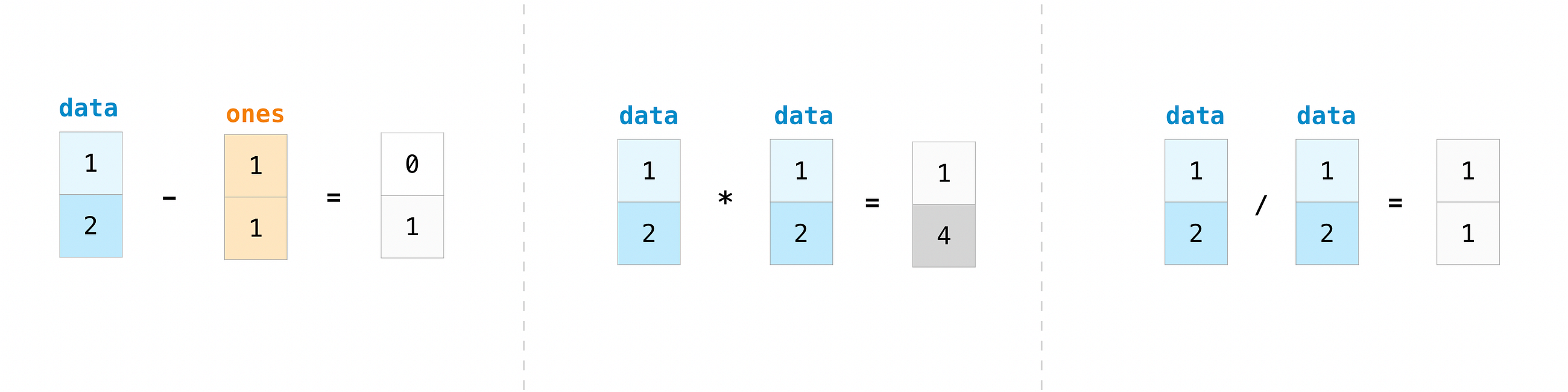Basic operations are simple with NumPy. If you want to find the sum of the elements in an array, you’d use sum(). This works for 1D arrays, 2D arrays, and arrays in higher dimensions.

Input:

``````a = np.array([1, 2, 3, 4])# Add all of the elements in the array
a.sum()``````

Output:

``10``

To add the rows or the columns in a 2D array, you would specify the axis.

Input:

``b = np.array([[1, 1], [2, 2]])``

You can sum the rows with:

Input:

``b.sum(axis=0)``

Output:

``array([3, 3])``

You can sum the columns with:

Input:

``b.sum(axis=1)``

Output:

``array([2, 4])``

There are times when you might want to carry out an operation between an array and a single number (also called an operation between a vector and a scalar) or between arrays of two different sizes. For example, your array (we’ll call it “data”) might contain information about distance in miles but you want to convert the information to kilometers. You can perform this operation with:

``data * 1.6``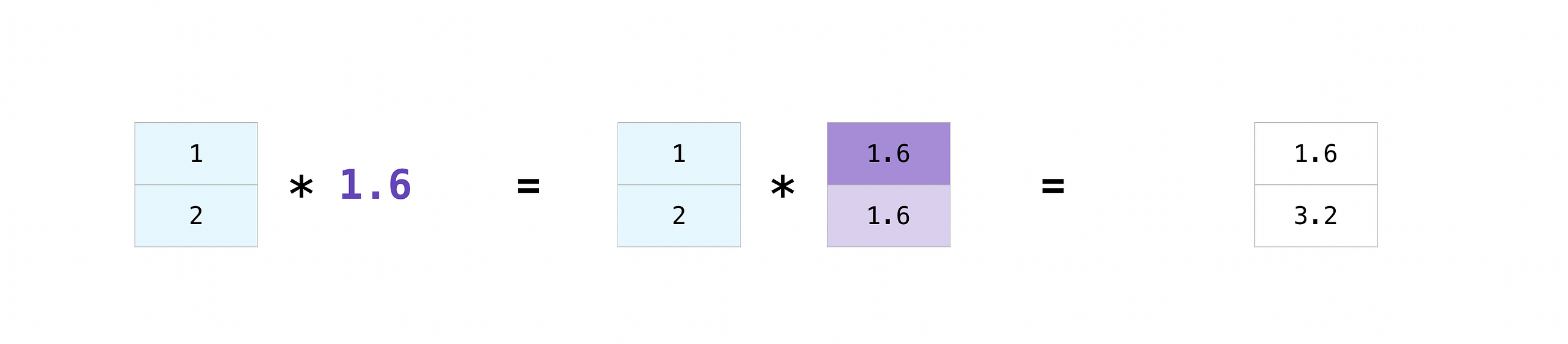NumPy understands that the multiplication should happen with each cell. That concept is called broadcasting. Broadcasting is a mechanism that allows NumPy to perform operations on arrays of different shapes. The dimensions of your array must be compatible, for example, when the dimensions of both arrays are equal or when one of them is 1. If the dimensions are not compatible, you will get a value error.

## More useful array operations

``Maximum, minimum, sum, mean, product, standard deviation, and more``

NumPy also performs aggregation functions. In addition to min, max, and sum, you can easily run mean to get the average, prod to get the result of multiplying the elements together, std to get the standard deviation, and more.

``````data.max()
data.min()
data.sum()``````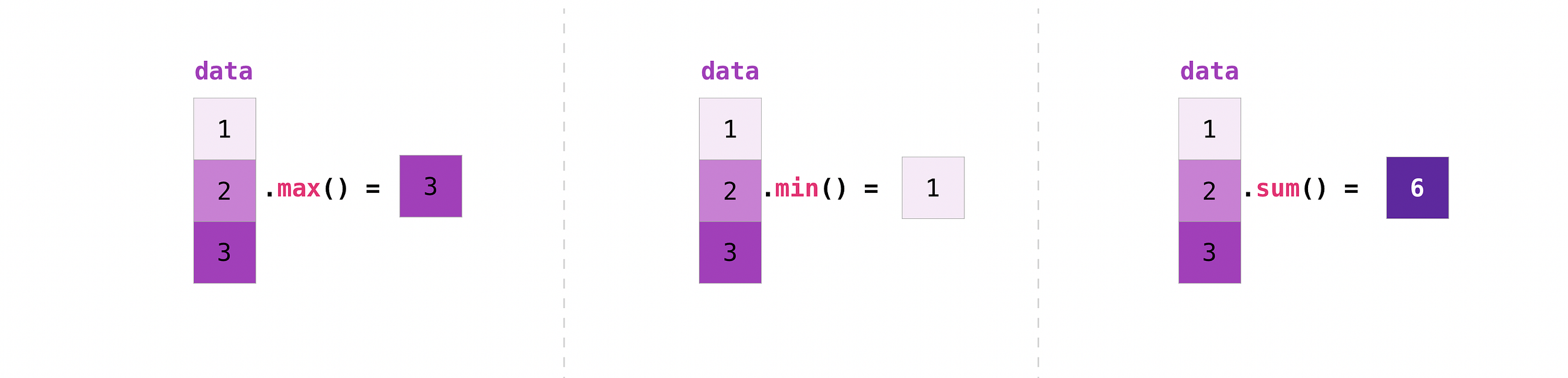``````[[0.45053314 0.17296777 0.34376245 0.5510652]
[0.54627315 0.05093587 0.40067661 0.55645993]
[0.12697628 0.82485143 0.26590556 0.56917101]]``````

It’s very common to want to aggregate along a row or column. By default, every NumPy aggregation function will return the aggregate of the entire array. To find the sum or the minimum of the elements in your array, run:

Input:

``A.sum()``

Or

``A.min()``

Output:

``````# Sum
4.8595783866706

# Minimum
0.050935870838424435``````

You can specify on which axis you want the aggregation function to be computed. For example, you can find the minimum value within each column by specifying axis=0.

Input:

``A.min(axis=0)``

Output:

``array([0.12697628, 0.05093587, 0.26590556, 0.5510652 ])``

The four values listed above correspond to the number of columns in your array. With a four-column array, you will get four values as your result.

## How to inspect the size and shape of a NumPy array

``````np.shape()
np.size()``````

You can get the dimensions of a NumPy array any time using ndarray.shape. NumPy will return the dimensions of the array as a tuple.

For example, if you create this array:

Input:

``np_arr = np.array([[1 , 2, 3, 4], [5, 6, 7, 8], [9, 10, 11, 12]])``

You can use np.shape to find the shape of your array.

Input:

``np_arr.shape``

Output:

``(3, 4)``

This output tells you that your array has three rows and four columns.

You can find just the number of rows by specifying :

Input:

``````num_of_rows = np_arr.shape

print('Number of Rows : ', num_of_rows)``````

Output:

``Number of Rows :  3``

Or just the number of columns by specifying :

Input:

``````num_of_columns = np_arr.shape

print('Number of Columns : ', num_of_columns)``````

Output:

``Number of Columns :  4``

It’s also easy to find the total number of elements in your array:

Input:

``print(np_arr.shape * np_arr.shape)``

Output:

``12``

You can use np.shape() with a 1D array as well. If you create this array:

Input:

``arr = np.array([1, 2, 3, 4, 5, 6, 7, 8])``

You can print the shape and the length of the array.

``````print('Shape of 1D array: ', arr.shape)
print('Length of 1D array: ', arr.shape)``````

Output:

``````Shape of 1D array:  (8,)
Length of 1D array:  8``````

You can get the dimensions of an array using np.size().

Input:

``````# get number of rows in array
num_of_rows2 = np.size(np_arr, 0)

# get number of columns in 2D numpy array
num_of_columns2 = np.size(np_arr, 1)

print('Number of Rows : ', num_of_rows2)
print('Number of Columns : ', num_of_columns2)``````

Output:

``````Number of Rows :  3
Number of Columns: 4``````

You can print the total number of elements as well:

Input:

``print('Total number of elements in  array : ', np.size(np_arr))``

Output:

``Total number of elements in  array :  12``

This also works for 3D arrays:

Input:

``````arr3D = np.array([ [[1, 1, 1, 1], [2, 2, 2, 2], [3, 3, 3, 3]],
[[4, 4, 4, 4], [5, 5, 5, 5], [6, 6, 6, 6]] ])``````

You can easily print the size of the axis:

Input:

``````print('Axis 0 size : ', np.size(arr3D, 0))
print('Axis 1 size : ', np.size(arr3D, 1))
print('Axis 2 size : ', np.size(arr3D, 2))``````

Output:

``````Axis 0 size :  2
Axis 1 size :  3
Axis 2 size :  4``````

You can print the total number of elements:

Input:

``print(np.size(arr3D))``

Output:

``24``

You can also use np.size() with 1D arrays:

Input:

``````# Create a 1D array
arr = np.array([1, 2, 3, 4, 5, 6, 7, 8])

# Determine the length
print('Length of 1D numpy array : ', np.size(arr))``````

Output:

``Length of 1D numpy array :  8``

Remember that if you check the size of your array and it equals 0, your array is empty.

Learn more about finding the size of an array here and the shape of an array here.

## Creating matrices

You can pass Python lists of lists to create a matrix to represent them in NumPy.

``np.array([[1,2],[3,4]])``Indexing and slicing operations are useful when you’re manipulating matrices:

``````data[0,1]
data[1:3]
data[0:2,0]``````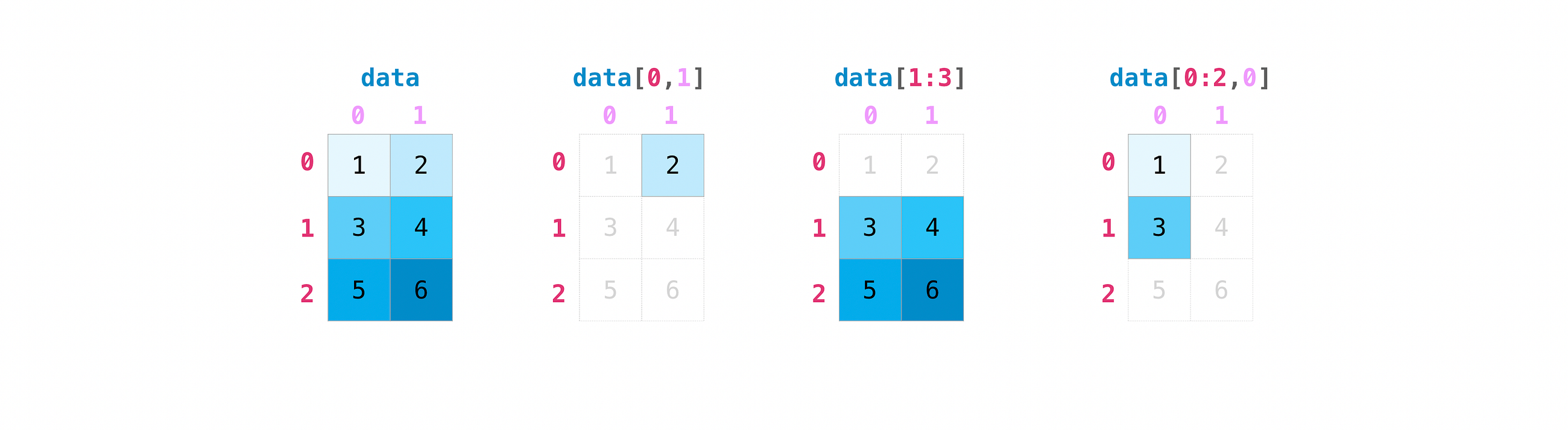You can aggregate matrices the same way you aggregated vectors:

``````data.max()
data.min()
data.sum()``````

You can aggregate all the values in a matrix and you can aggregate them across columns or rows using the axis parameter:

``````data.max(axis=0)
data.max(axis=1)``````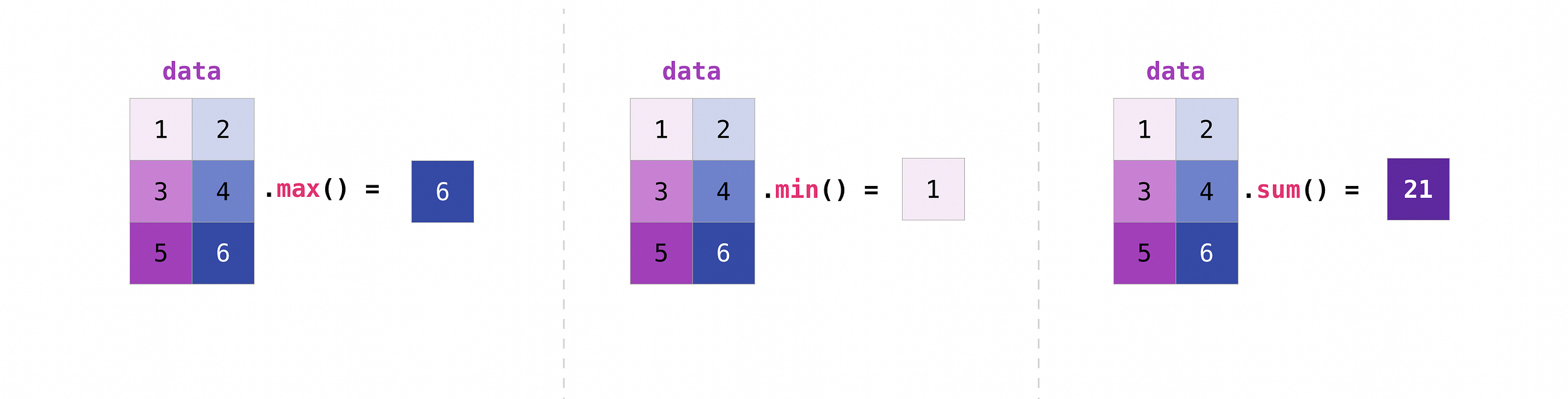Once you’ve created your matrices, you can add and multiply them using arithmetic operators if you have two matrices that are the same size.

``data + ones``You can do these arithmetic operations on matrices of different sizes, but only if one matrix has only one column or one row. In this case, NumPy will use its broadcast rules for the operation.

``data + ones_row``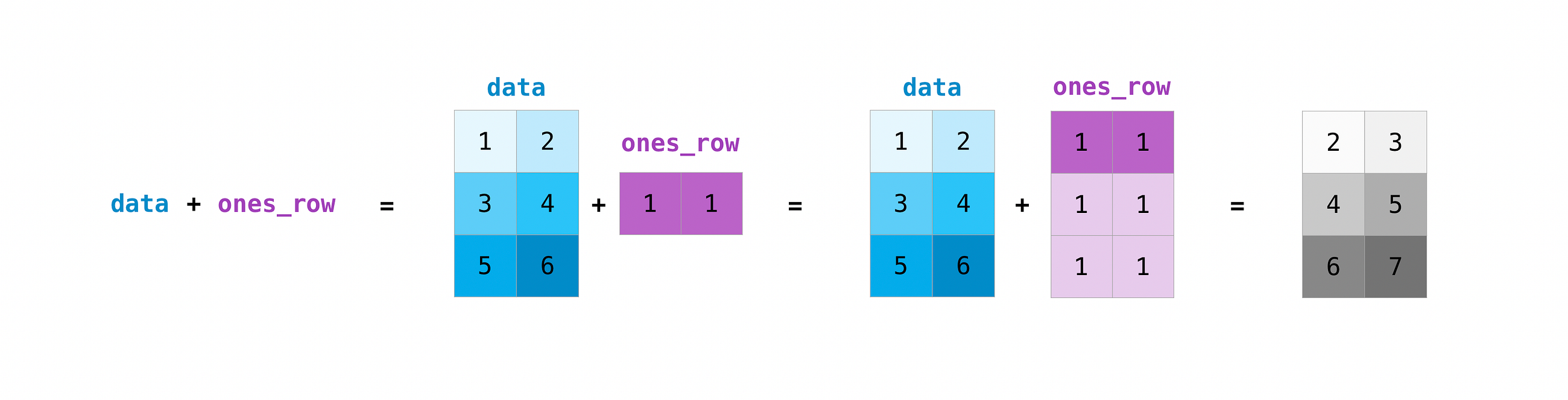Be aware that when NumPy prints N-Dimensional arrays, the last axis is looped over the fastest while the first axis is the slowest. That means that:

Input:

``np.ones((4,3,2))``

Will print out like this:

Output:

``````array([[[1., 1.],
[1., 1.],
[1., 1.]],     [[1., 1.],
[1., 1.],
[1., 1.]],     [[1., 1.],
[1., 1.],
[1., 1.]],     [[1., 1.],
[1., 1.],
[1., 1.]]])``````

There are often instances where we want NumPy to initialize the values of an array. NumPy offers methods like ones(), zeros(), and random.random() for these instances. All you need to do is pass in the number of elements you want it to generate.

``````np.ones(3)
mp.zeros(3)
np.random.random((3)``````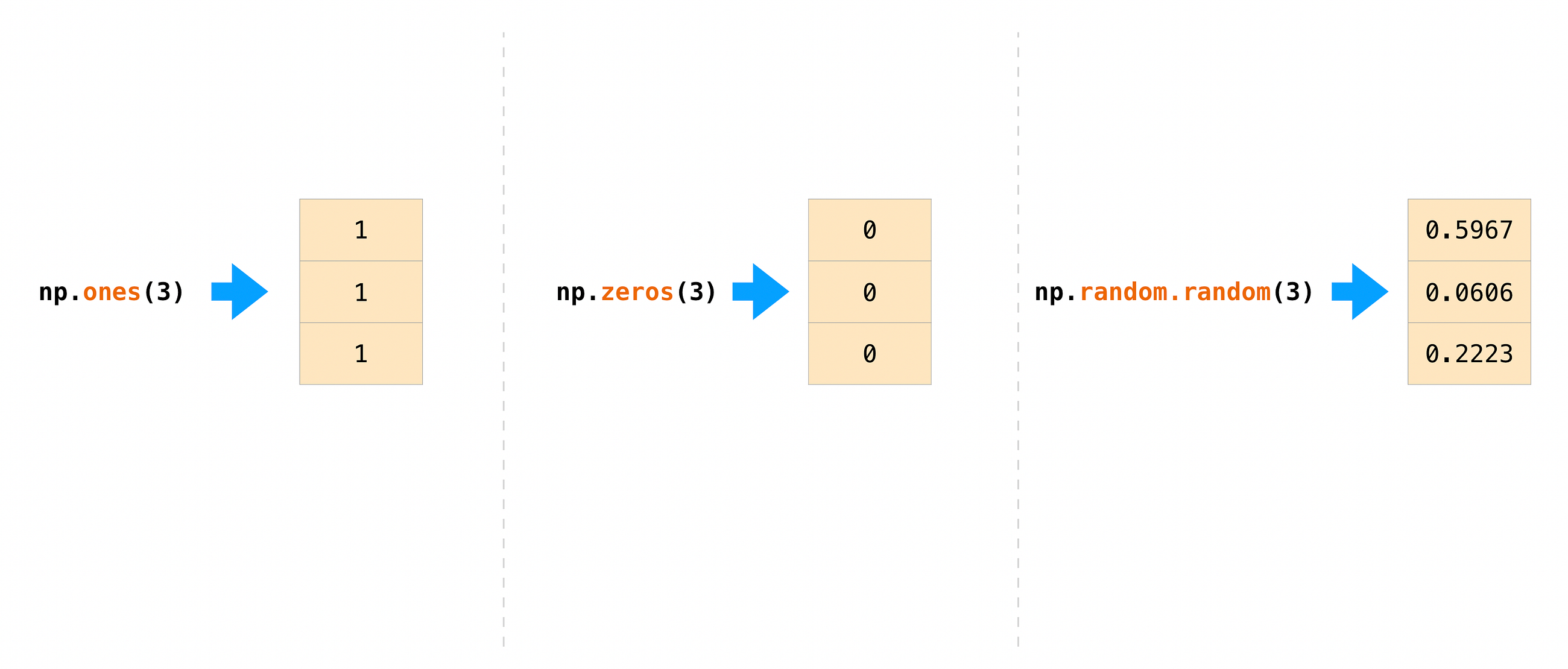Read more about initializing the values of an array with ones here, zeros here, and initializing empty arrays here.

## Generating random numbers

The use of random number generation is an important part of the configuration and evaluation of machine learning algorithms. Whether you need to randomly initialize weights in an artificial neural network, split data into random sets, or randomly shuffle your dataset, being able to generate random numbers (actually, repeatable pseudo-random numbers) is essential.

You have a number of options when using NumPy for random number generation. Random Generator is NumPy’s replacement for RandomState. The main difference between them is that Generator relies on an additional BitGenerator to manage state and generate the random bits, which are transformed into random values.

With Generator.integers, you can generate random integers from low (remember that this is inclusive with NumPy) to high (exclusive). You can set endopoint=True to make the high number inclusive.

You can generate a 2 x 4 array of random integers between 0 and 4 with

Input:

``rng.integers(5, size=(2, 4))``

Output:

``````array([[4, 0, 2, 1],
[3, 2, 2, 0]])``````

You can also use the ones(), zeros(), and random() methods to create an array if you give them a tuple describing the dimensions of the matrix.

``````np.ones(3,2)
mp.zeros(3,2)
np.random.random((3,2)``````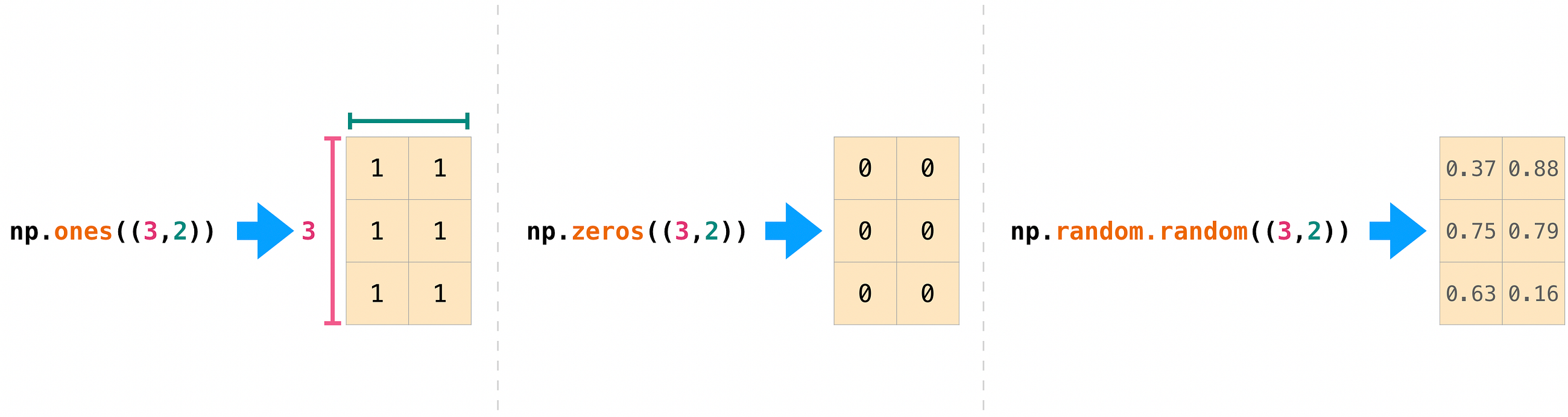## How to get unique items and counts

``np.unique()``

You can find the unique elements in an array easily with np.unique.

Input:

``a = np.array([11, 11, 12, 13, 14, 15, 16, 17, 12, 13, 11, 14, 18, 19, 20])``

you can use np.unique

Input:

``````unique_values = np.unique(a)
print(unique_values)``````

Output:

``[11 12 13 14 15 16 17 18 19 20]``

To get the indices of unique values in a NumPy array (an array of first index positions of unique values in the array), just pass the return_index argument in np.unique() as well as your array.

Input:

``````indices_list = np.unique(a, return_index=True)
print(indices_list)``````

Output:

``[ 0  2  3  4  5  6  7 12 13 14]``

You can pass the `return_counts` argument in np.unique() along with your array to get the frequency count of unique values in a NumPy array.

Input:

``````unique_values, occurrence_count = np.unique(a, return_counts=True)
print(occurrence_count)``````

Output:

``[3 2 2 2 1 1 1 1 1 1]``

This also works with 2D arrays. If you start with this array:

``a2D = np.array([[1, 2, 3, 4] ,[5, 6, 7, 8] , [9, 10, 11, 12],  [1, 2, 3, 4]])``

You can find unique values with:

Input:

``````unique_values = np.unique(a2D)
print(unique_values)``````

Output:

``[ 1  2  3  4  5  6  7  8  9 10 11 12]``

If the axis argument isn’t passed, your 2D array will be flattened.

To get the unique rows or columns, make sure to pass the axis argument. To find the unique rows, specify `axis=0` and for columns, specify `axis=1`.

Input:

``````unique_rows = np.unique(a2D, axis=0)
print(unique_rows)``````

Output:

``````[[ 1  2  3  4]
[ 5  6  7  8]
[ 9 10 11 12]]``````

To get the unique rows, occurrence count, and index position, you can use:

Input:

``````unique_rows, occurence_count, indices = np.unique(a2D, axis=0, return_counts=True, return_index=True)
print('Unique Rows: ', '\n', unique_rows)
print('Occurrence Count:', '\n', occurence_count)
print('Indices: ', '\n', indices)``````

Output:

``````Unique Rows:
[[ 1  2  3  4]
[ 5  6  7  8]
[ 9 10 11 12]]
Occurrence Count:
[0 1 2]
Indices:
[2 1 1]``````

## Transposing and reshaping a matrix

``````np.reshape()
np.transpose()
np.T()``````

It’s common to need to rotate your matrices. NumPy arrays have the property T that allows you to transpose a matrix.You may also need to switch the dimensions of a matrix. This can happen when, for example, you have a model that expects a certain input shape that is different from your dataset. This is where the reshape method can be useful. You simply need to pass in the new dimensions that you want for the matrix.

``````data.reshape(2,3)
data.reshape(3,2)``````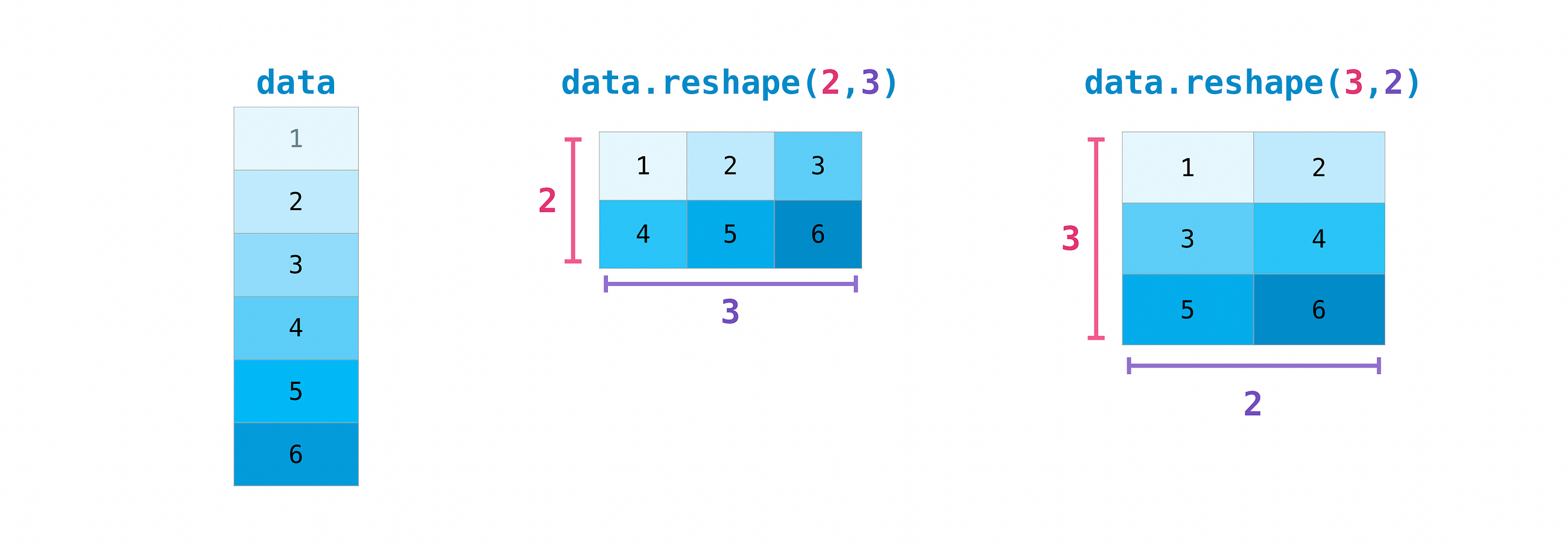You can also use np.transpose to reverse or change the axes of an array according to the values you specify.

``````arr = np.arange(6).reshape((2,3))
arr``````

Output:

``````array([[0, 1, 2],
[3, 4, 5]])``````

You can transpose your array with np.transpose().

Input:

``np.transpose(arr)``

Output:

``````array([[0, 3],
[1, 4],
[2, 5]])``````

## How to reverse an array

``np.flip``

NumPy’s np.flip() function allows you to flip, or reverse, the contents of an array along an axis. When using np.flip, specify the array you would like to reverse and the axis. If you don’t specify the axis, NumPy will reverse the contents along all of the axes of your input array.

Reversing a 1D array

If you begin with a 1D array like this one:

``arr = np.array([1, 2, 3, 4, 5, 6, 7, 8])``

You can reverse it with:

``reversed_arr = np.flip(arr)``

If you want to print your reversed array, you can run:

Input:

``print('Reversed Array: ', reversed_arr)``

Output:

``Reversed Array:  [8 7 6 5 4 3 2 1]``

Reversing a 2D array

A 2D array works much the same way.

Input:

``arr2D = np.array([[1 , 2, 3, 4], [5, 6, 7, 8], [9, 10, 11, 12]])``

You can reverse the content in all of the rows and all of the columns with:

Input:

``````reversed_arr = np.flip(arr2D)

print('Reversed Array: ')
print(reversed_arr)``````

Output:

``````Reversed Array:
[[12 11 10  9]
[ 8  7  6  5]
[ 4  3  2  1]]``````

You can easily reverse only the rows with:

Input:

``````reversed_arr_rows = np.flip(arr2D, axis=0)

print('Reversed Array: ')
print(reversed_arr_rows)``````

Output:

``````Reversed Array:
[[ 9 10 11 12]
[ 5  6  7  8]
[ 1  2  3  4]]``````

Or reverse only the columns with:

Input:

``````reversed_arr_columns = np.flip(arr2D, axis=1)

print('Reversed Array columns: ')
print(reversed_arr_columns)``````

Output:

``````Reversed Array columns:
[[ 4  3  2  1]
[ 8  7  6  5]
[12 11 10  9]]``````

You can also reverse the contents of only one column or row. For example, you can reverse the contents of the row at index position 1 (the second row):

Input:

``````arr2D = np.flip(arr2D)

print('Reversed Array: ')
print(arr2D)``````

Output:

``````Reversed Array:
[[ 1  2  3  4]
[ 5  6  7  8]
[ 9 10 11 12]]``````

You can also reverse the column at index position 1 (the second column):

Input:

``````arr2D[:,1] = np.flip(arr2D[:,1])

print('Reversed Array: ')
print(arr2D)``````

Output:

``````Reversed Array:
[[ 1 10  3  4]
[ 5  6  7  8]
[ 9  2 11 12]]``````

## Reshaping and flattening multidimensional arrays

``````.flatten()
.ravel()``````

There are two popular ways to flatten an array: .flatten() and .ravel(). The primary difference between the two is that the new array created using ravel() is actually a reference to the parent array. This means that any changes to the new array will affect the parent array as well. Since ravel does not create a copy, it’s memory efficient.

``array = np.array([[1 , 2, 3, 4], [5, 6, 7, 8], [9, 10, 11, 12]])``

You can use flatten to flatten your array into a 1D array.

Input:

``array.flatten()``

Output:

``array([ 1,  2,  3,  4,  5,  6,  7,  8,  9, 10, 11, 12])``

When you use flatten, changes to your new array won’t change the parent array.

For example:

Input:

``````a1 = array.flatten()
a1 = 100
print('Original array: ')
print(array)
print('New array: ')
print(a1)``````

Output:

``````Original array:
[[ 1  2  3  4]
[ 5  6  7  8]
[ 9 10 11 12]]
New array:
[100   2   3   4   5   6   7   8   9  10  11  12]``````

But when you use rank, the changes you make to the new array will affect the parent array.

For example:

Input:

``````a2 = array.ravel()
a2 = 101
print('Original array: ')
print(array)
print('New array: ')
print(a2)``````

Output:

``````Original array:
[[101   2   3   4]
[  5   6   7   8]
[  9  10  11  12]]
New array:
[101   2   3   4   5   6   7   8   9  10  11  12]``````

``````help()
?
??``````

When it comes to the data science ecosystem, Python and NumPy are built with the user in mind. One of the best examples of this is the built-in access to documentation. Every object contains the reference to a string, which is known as the docstring. In most cases, this docstring contains a quick and concise summary of the object and how to use it. Python has a built-in help() function that can help you access this information. This means that nearly any time you need more information, you can use help() to quickly find the information that you need.

For example,

``help(max)``

Will return

``````Help on built-in function max in module builtins:

max(...)
max(iterable, *[, default=obj, key=func]) -> value
max(arg1, arg2, *args, *[, key=func]) -> value    With a single iterable argument, return its biggest item. The
default keyword-only argument specifies an object to return if
the provided iterable is empty.
With two or more arguments, return the largest argument.``````

Because access to additional information is so useful, IPython uses the ? character as a shorthand for accessing this documentation along with other relevant information.

For example,

``max?``

Will return

``````Docstring:
max(iterable, *[, default=obj, key=func]) -> value
max(arg1, arg2, *args, *[, key=func]) -> valueWith a single iterable argument, return its biggest item. The
default keyword-only argument specifies an object to return if
the provided iterable is empty.
With two or more arguments, return the largest argument.
Type:      builtin_function_or_method``````

You can even use this notation for object methods and objects themselves.

Let’s say you create this array:

``a = np.array([1, 2, 3, 4, 5, 6])``

Running

``a?``

Will return a lot of useful information.

``````Type:            ndarray
String form:     [1 2 3 4 5 6]
Length:          6
File:            ~/anaconda3/lib/python3.7/site-packages/numpy/__init__.py
Docstring:       <no docstring>
Class docstring:
ndarray(shape, dtype=float, buffer=None, offset=0,
strides=None, order=None)An array object represents a multidimensional, homogeneous array
of fixed-size items.  An associated data-type object describes the
format of each element in the array (its byte-order, how many bytes it
occupies in memory, whether it is an integer, a floating point number,
or something else, etc.)Arrays should be constructed using `array`, `zeros` or `empty` (refer
to the See Also section below).  The parameters given here refer to
a low-level method (`ndarray(...)`) for instantiating an array.For more information, refer to the `numpy` module and examine the
methods and attributes of an array.Parameters
----------
(for the __new__ method; see Notes below)shape : tuple of ints
Shape of created array.
dtype : data-type, optional
Any object that can be interpreted as a numpy data type.
buffer : object exposing buffer interface, optional
Used to fill the array with data.
offset : int, optional
Offset of array data in buffer.
strides : tuple of ints, optional
Strides of data in memory.
order : {'C', 'F'}, optional
Row-major (C-style) or column-major (Fortran-style) order.Attributes
----------
T : ndarray
Transpose of the array.
data : buffer
The array's elements, in memory.
dtype : dtype object
Describes the format of the elements in the array.
flags : dict
Dictionary containing information related to memory use, e.g.,
'C_CONTIGUOUS', 'OWNDATA', 'WRITEABLE', etc.
flat : numpy.flatiter object
Flattened version of the array as an iterator.  The iterator
allows assignments, e.g., ``x.flat = 3`` (See `ndarray.flat` for
assignment examples; TODO).
imag : ndarray
Imaginary part of the array.
real : ndarray
Real part of the array.
size : int
Number of elements in the array.
itemsize : int
The memory use of each array element in bytes.
nbytes : int
The total number of bytes required to store the array data,
i.e., ``itemsize * size``.
ndim : int
The array's number of dimensions.
shape : tuple of ints
Shape of the array.
strides : tuple of ints
The step-size required to move from one element to the next in
memory. For example, a contiguous ``(3, 4)`` array of type
``int16`` in C-order has strides ``(8, 2)``.  This implies that
to move from element to element in memory requires jumps of 2 bytes.
To move from row-to-row, one needs to jump 8 bytes at a time
(``2 * 4``).
ctypes : ctypes object
Class containing properties of the array needed for interaction
with ctypes.
base : ndarray
If the array is a view into another array, that array is its `base`
(unless that array is also a view).  The `base` array is where the
--------
array : Construct an array.
zeros : Create an array, each element of which is zero.
empty : Create an array, but leave its allocated memory unchanged (i.e.,
it contains "garbage").
dtype : Create a data-type.Notes
-----
There are two modes of creating an array using ``__new__``:1. If `buffer` is None, then only `shape`, `dtype`, and `order`
are used.
2. If `buffer` is an object exposing the buffer interface, then
all keywords are interpreted.No ``__init__`` method is needed because the array is fully initialized
after the ``__new__`` method.Examples
--------
These examples illustrate the low-level `ndarray` constructor.  Refer
to the `See Also` section above for easier ways of constructing an
ndarray.First mode, `buffer` is None:>>> np.ndarray(shape=(2,2), dtype=float, order='F')
array([[ -1.13698227e+002,   4.25087011e-303],
[  2.88528414e-306,   3.27025015e-309]])         #randomSecond mode:>>> np.ndarray((2,), buffer=np.array([1,2,3]),
...            offset=np.int_().itemsize,
...            dtype=int) # offset = 1*itemsize, i.e. skip first element
array([2, 3])``````

This also works for functions and other objects that you create. Just remember to include a docstring with your function using a string literal (“““ “”” or ‘’’ ‘’’ around your documentation).

For example, if you create this function:

``````def double(a):
'''Return a * 2'''
return a * 2``````

You can run:

``double?``

Which will return:

``````Signature: double(a)
Docstring: Return a * 2
Type:      function``````

You can reach another level of information by reading the source code of the object you’re interested in. Using a double question mark (??) allows you to access the source code.

For example, running:

``double??``

Will return:

``````Signature: double(a)
Source:
def double(a):
'''Return a * 2'''
return a * 2
Type:      function``````

If the object in question is compiled in a language other than Python, using ?? will return the same information as ?. You’ll find this with a lot of built-in objects and types, for example:

``len?``

Output:

``````Signature: len(obj, /)
Docstring: Return the number of items in a container.
Type:      builtin_function_or_method``````

and

``len??``

Output:

``````​Signature: len(obj, /)
Docstring: Return the number of items in a container.
Type:      builtin_function_or_method``````

Have the same output because they were compiled in a programming language other than Python.

## Working with mathematical formulas

Implementing mathematical formulas that work on arrays is one of the things that make NumPy so highly regarded in the scientific Python community.

For example, this is the mean square error formula (a central formula used in supervised machine learning models that deal with regression):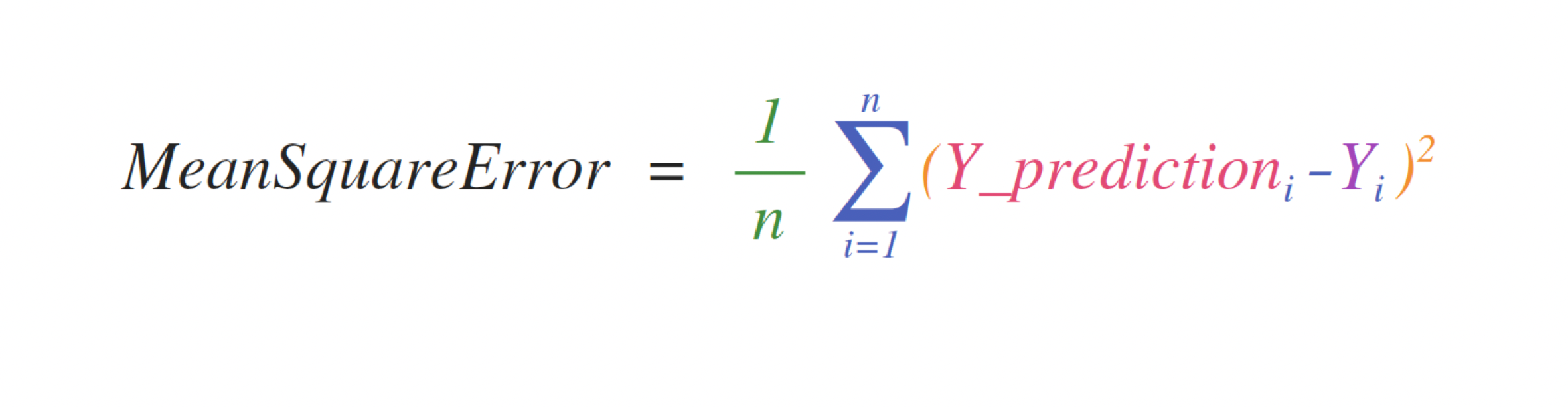Implementing this formula is simple and straightforward in NumPy: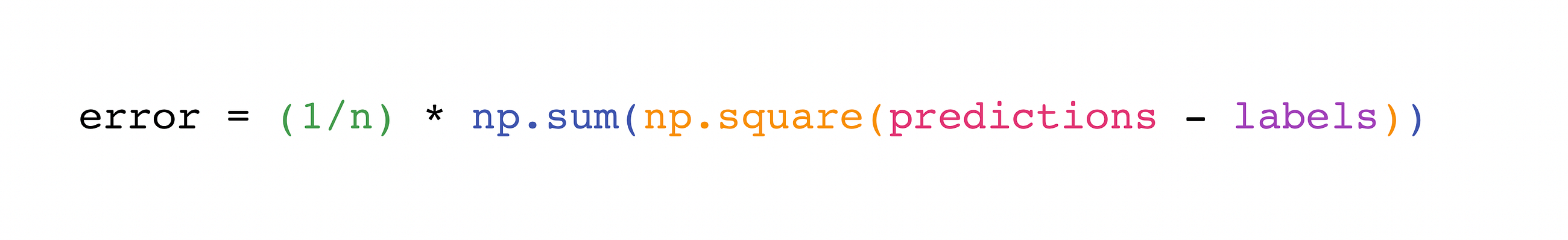What makes this work so well is that predictions and labels can contain one or a thousand values. They only need to be the same size.

You can visualize it this way: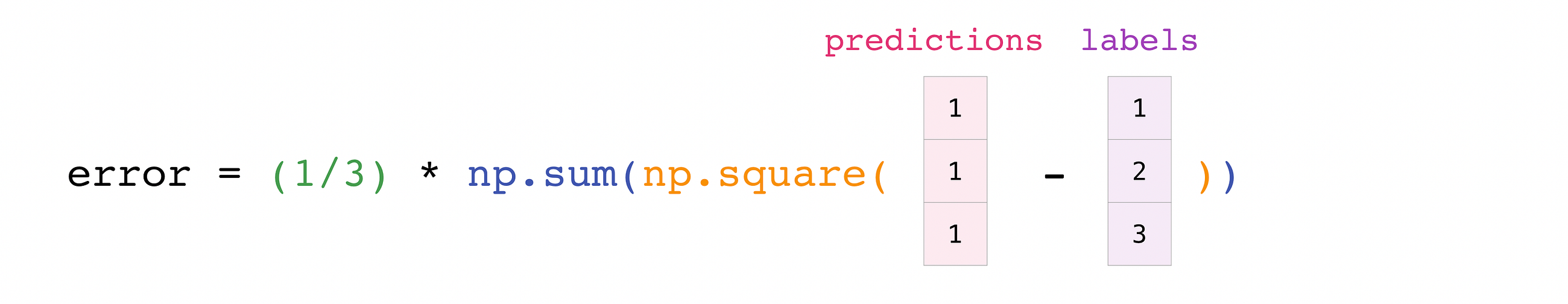In this example, both the predictions and labels vectors contain three values, meaning n has a value of three. After we carry out subtractions the values in the vector are squared. Then NumPy sums the values, and your result is the error value for that prediction and a score for the quality of the model.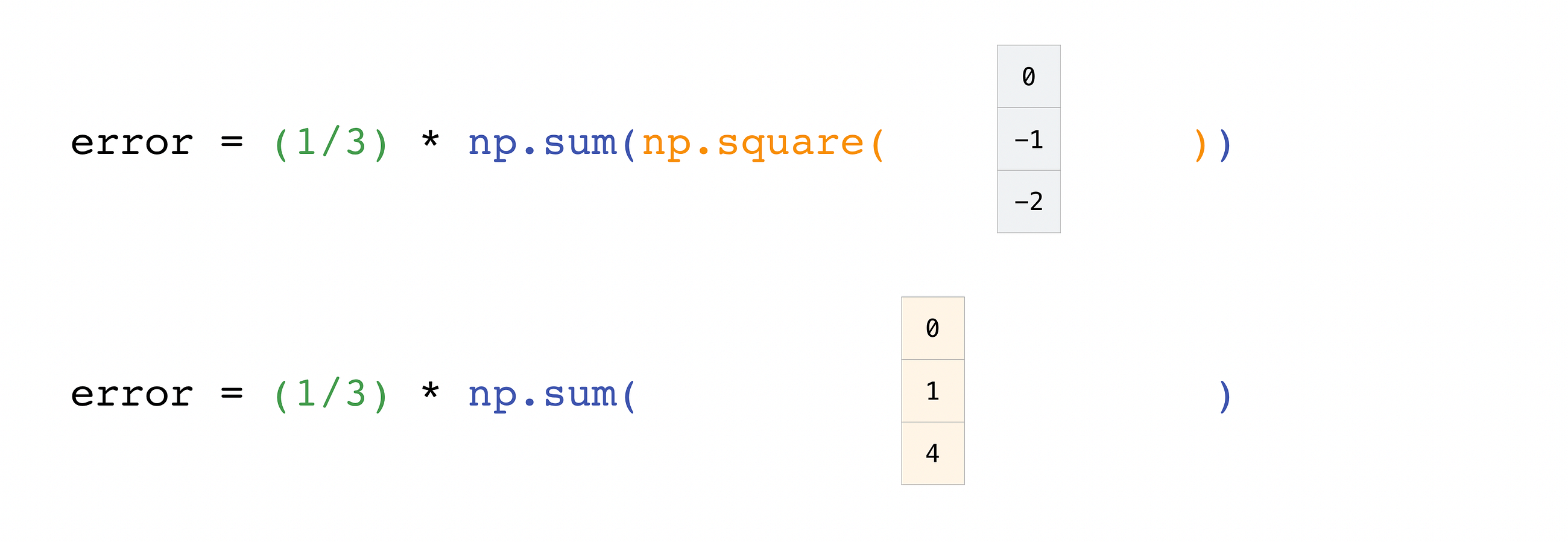## How to save and load NumPy objects

``````np.save()
np.savez()
np.savetxt()

You will, at some point, want to save your arrays to disk and load them back without having to re-run the code. Fortunately, there are several ways to save and load objects with Numpy. The ndarray objects can be saved to and loaded from the disk files with loadtxt and savetxt functions that handle normal text files, load and save functions that handle NumPy binary files with a .npy file extension, and a savez function that handles NumPy files with a .npz file extension.

The .npy and .npz files store data, shape, dtype, and other information required to reconstruct the ndarray in a way that allows the array to be correctly retrieved, even when the file is on another machine with different architecture.

If you want to store a single ndarray object, store it as a .npy file using np.save. If you want to store more than one ndarray object in a single file, save it as a .npz file using np.savez. You can also save several arrays into a single file in compressed npz format with np.savez_compressed.

It’s easy to save and load and array with np.save(). Just make sure to specify the array you want to save and a file name. For example, if you create this array:

``a = np.array([1, 2, 3, 4, 5, 6])``

You can save it as “filename.npy” with

``np.save('filename',a)``

``b = np.load('filename.npy')``

If you want to check your array, you can run:

Input:

``print(b)``

Output:

``[1 2 3 4 5 6]``

You can save a NumPy array as a plain text file like a .csv or .txt file with np.savetxt.

For example, if you create this array:

``csv_arr = np.array([1, 2, 3, 4, 5, 6, 7, 8])``

You can easily save it as a .csv file with the name “new_file.csv” like this:

``np.savetxt('new_file.csv', csv_arr)``

Input:

``np.loadtxt('new_file.csv')``

Output:

``array([1., 2., 3., 4., 5., 6., 7., 8.])``

The savetxt() and loadtxt() functions accept additional optional parameters such as header, footer, and delimiter. While text files can be easier for sharing, .npy and .npz files are faster to retrieve. If you need more sophisticated handling of your text file (for example, if you need to work with lines that contain missing values), you will want to use the genfromtxt function.

Be aware that loading files that contain object arrays with np.load() uses the pickle module which is not secure against erroneous or maliciously constructed data. Consider passing `allow_pickle=False` to load data that is known not to contain object arrays for the safer handling of untrusted sources.

## Importing and exporting a CSV

It’s simple to read in a CSV that contains existing information. The best and easiest way to do this is to use Pandas.

``````import pandas as pd# If all of your columns are the same type:
x = pd.read_csv('music.csv').values# You can also simply select the columns you need: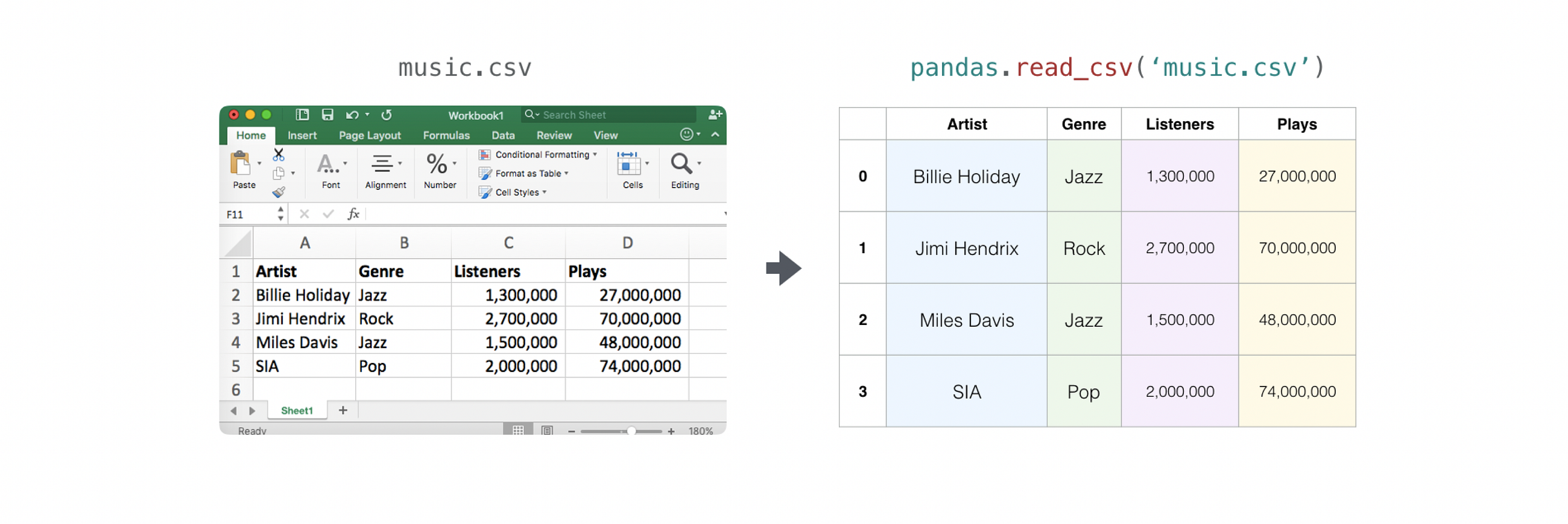It’s simple to use Pandas in order to export your array as well. If you are new to NumPy, you may want to create a Pandas dataframe from the values in your array and then write the data frame to a CSV file with Pandas.

If you created this array “a”

``````[[-2.58289208,  0.43014843, -1.24082018,  1.59572603],
[ 0.99027828,  1.17150989,  0.94125714, -0.14692469],
[ 0.76989341,  0.81299683, -0.95068423,  0.11769564],
[ 0.20484034,  0.34784527,  1.96979195,  0.51992837]]``````

You could create a Pandas dataframe

``````df = pd.DataFrame(a)
print(df)``````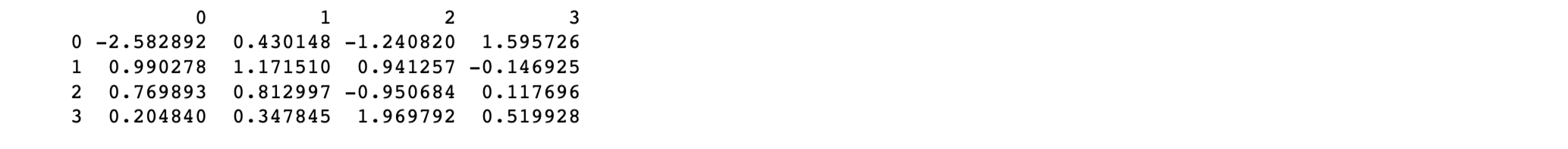You can easily save your dataframe with

``df.to_csv('pd.csv')``

``pd.read_csv('pd.csv')``You can also save your array with the NumPy “savetxt” method.

``np.savetxt('np.csv', a, fmt='%.2f', delimiter=',', header=" 1,  2,  3,  4")``

Input:

``cat np.csv``

Output:

``````#  1,  2,  3,  4
-2.58,0.43,-1.24,1.60
0.99,1.17,0.94,-0.15
0.77,0.81,-0.95,0.12
0.20,0.35,1.97,0.52``````

If you’re interested in learning more about Pandas, take a look at the official Pandas website. Learn how to install Pandas with the official Pandas installation information.

## Plotting arrays with Matplotlib

If you need to generate a plot for your values, it’s very simple with Matplotlib.

For example, you may have an array like this one:

``A = np.array([2, 1, 5, 7, 4, 6, 8, 14, 10, 9, 18, 20, 22])``

If you already have Matplotlib installed, you can import it with

``````import matplotlib.pyplot as plt
# If you're using Jupyter Notebook, you may also want to run the following line of code
to display your code in the notebook
%matplotlib inline``````

All you need to do to plot your values is run

Input:

``````plt.plot(A)
plt.show()``````

Output: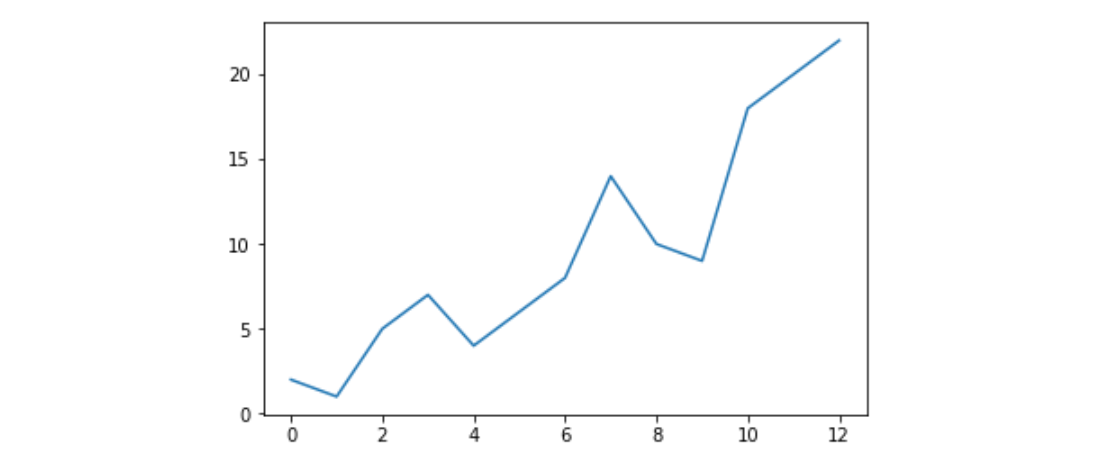For example, you can plot a 1D array like this:

Input:

``````x = np.linspace(0, 5, 20)
y = np.linspace(0, 10, 20)
plt.plot(x, y, 'purple') # line
plt.plot(x, y, 'o')      # dots``````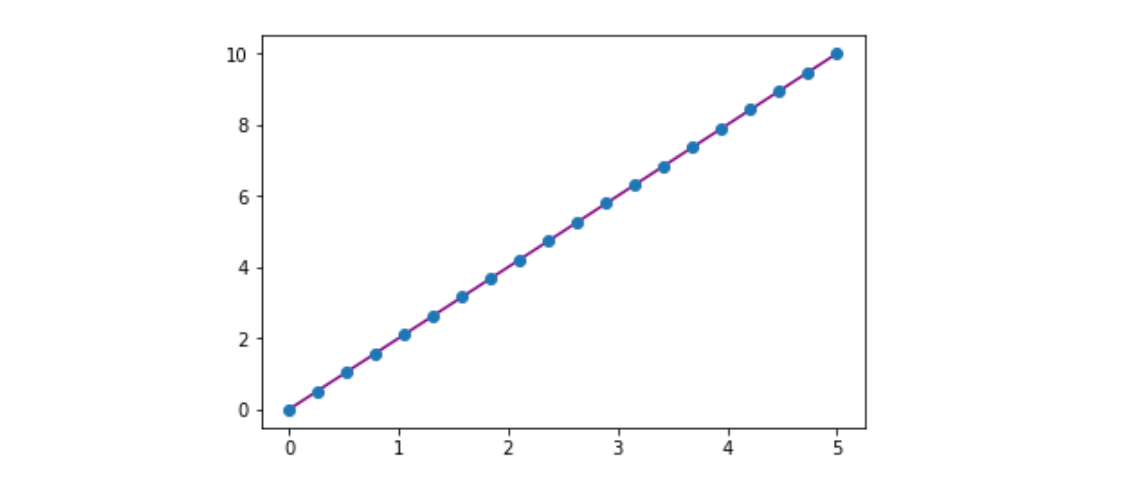With Matplotlib, you have access to an enormous number of visualization options.

``````from mpl_toolkits.mplot3d import Axes3Dfig = plt.figure()
ax = Axes3D(fig)
X = np.arange(-5, 5, 0.15)
Y = np.arange(-5, 5, 0.15)
X, Y = np.meshgrid(X, Y)
R = np.sqrt(X**2 + Y**2)
Z = np.sin(R)ax.plot_surface(X, Y, Z, rstride=1, cstride=1, cmap='viridis')plt.colorbar()``````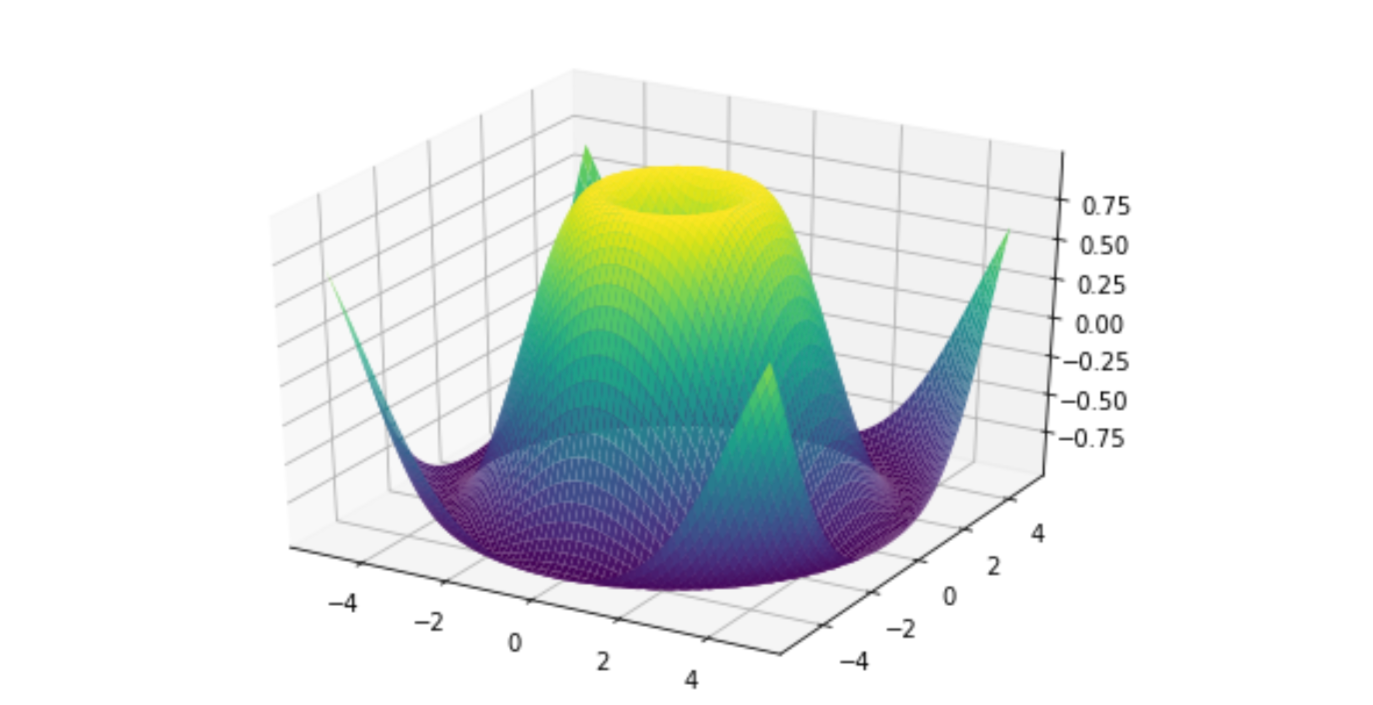To read more about Matplotlib and what it can do, take a look at the official documentation. For directions regarding installing Matplotlib, see the official installation section.## Data Science Projects | Data Science | Machine Learning | Python

Practice your skills in Data Science with Python, by learning and then trying all these hands-on, interactive projects, that I have posted for you.

## Data Science Projects | Data Science | Machine Learning | Python

Practice your skills in Data Science with Python, by learning and then trying all these hands-on, interactive projects, that I have posted for you.

## Data Science Projects | Data Science | Machine Learning | Python

Practice your skills in Data Science with Python, by learning and then trying all these hands-on, interactive projects, that I have posted for you.

## Data Science Projects | Data Science | Machine Learning | Python

Practice your skills in Data Science with Python, by learning and then trying all these hands-on, interactive projects, that I have posted for you.

## Data Science Projects | Data Science | Machine Learning | Python

Practice your skills in Data Science with Python, by learning and then trying all these hands-on, interactive projects, that I have posted for you.